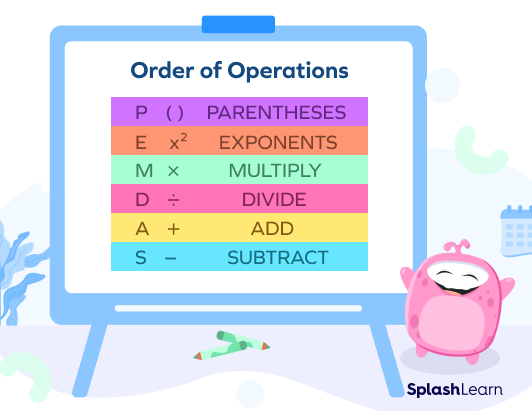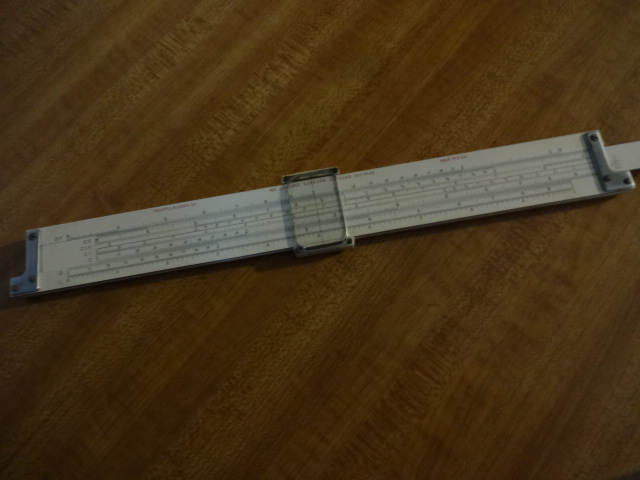# Simple Math - Right?

Youse guys have fun with this math equation. My math tutor just sent me one to solve…3 Likes

This tutor is just giving hints:6 Likes

My calculator says the answer is 1…so the correct answer is 1 no matter how someone tries to rationalize it…go argue with casio fx-9860G II

1 Like

Which math problem above are you referring to? I haven’t commented, other than posting a Youtube link about the “6 ÷ 2(1 + 2)”.1 Like
3 Likes

LOL…yes…that’s the one…the video said a hundred years ago the answer was 1 but now it’s 9…but casio says 1…so that’s it…

2 Likes

It all depends on how you key it into the calculator. 6 / 2 x (1+2) always returns 9. Parentheses first and then left to right as division and multiplication have equal precedence.
If you key it in as written 6 / 2(1+2) you will get a 1. This is because the entire right expression 2(1+2) is completed first so you wind up with 6 / 6 which is 1.

Curiously while the above holds true for my handheld calculators (Casio - TI) The windows scientific calculator answers both entry methods with a 9.

My Real Calc app calculator on my phone returns 9 for either entry method but Bit Calc app will not recognize an implied operation (2(1+2)) so it returns a 2 (6 / 3).

1 Like

LOL…windows…if we’ve learn anything about the past couple of years…is that computers cannot be trusted to count…correctly…and now we know why…

1 Like
3 Likes

Is this that “new math” I’ve been hearing about?1 Like

The rule is that multiplication and division have the same precedence (as do addition and subtraction). Therefore operations occur from left to right. Watch the answer video for a complete explanation. As I explained above, if you key it in the calculator and specify the multiplication, as opposed to expecting the calculator to know, you will come out with a 9.
I am confident this will be the case with your Casio fx-9860G II. Try it.

1 Like

I got 9 on my K & E. What’s a Casio?3 Likes
1 Like